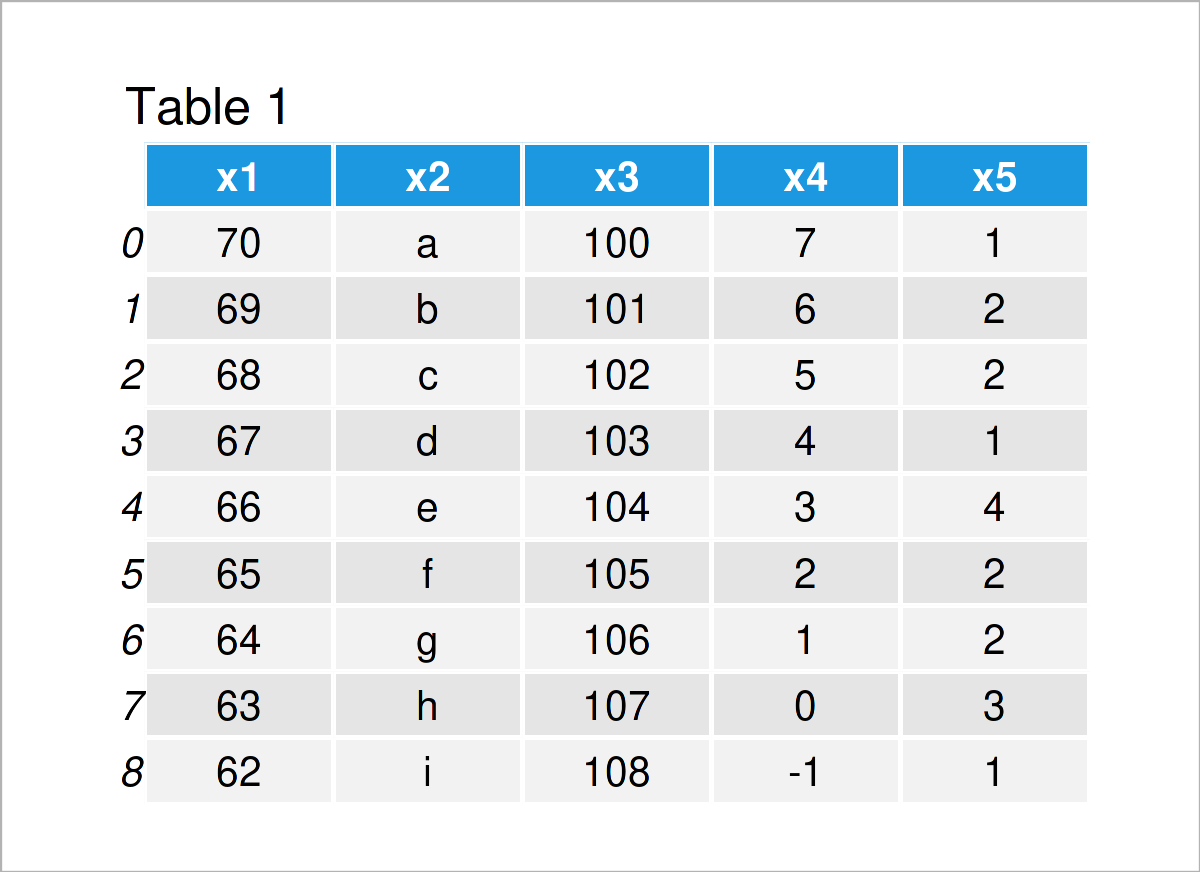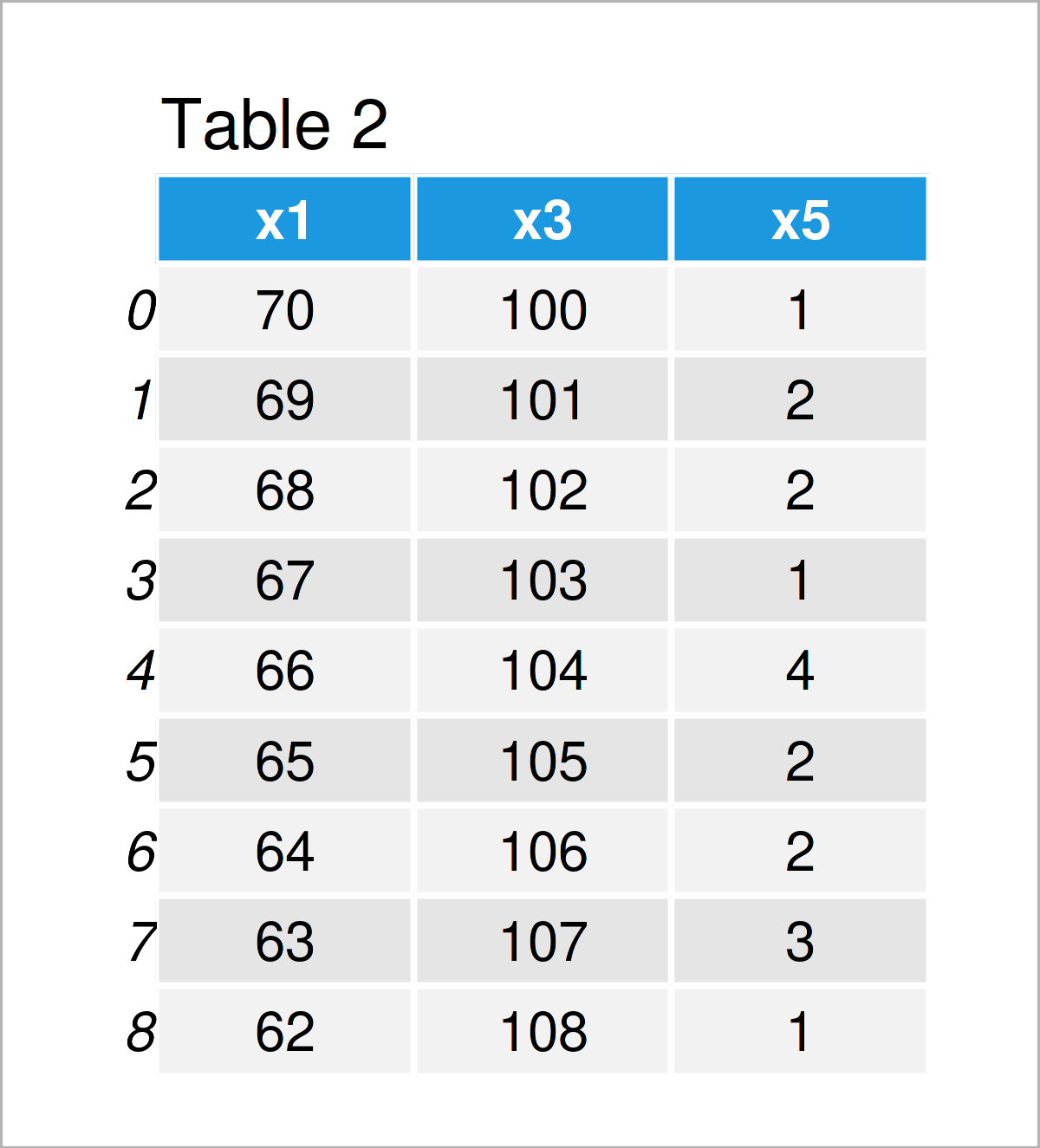# Create Subset of Columns of pandas DataFrame in Python (Example)

This tutorial shows how to extract a subset of columns of a pandas DataFrame in the Python programming language.

The tutorial contains the following:

Let’s dig in:

## Exemplifying Data & Add-On Libraries

If we want to use the functions of the pandas library, we first need to import pandas:

`import pandas as pd                              # Import pandas library to Python`

As a next step, let’s also create some example data:

```data = pd.DataFrame({'x1':range(70, 61, - 1),    # Create pandas DataFrame
'x2':['a', 'b', 'c', 'd', 'e', 'f', 'g', 'h', 'i'],
'x3':range(100, 109),
'x4':range(7, - 2, - 1),
'x5':[1, 2, 2, 1, 4, 2, 2, 3, 1]})
print(data)                                      # Print pandas DataFrame```Table 1 shows that our example pandas DataFrame consists of nine rows and five variables.

## Example: Extract Subset of Columns in pandas DataFrame

In this example, I’ll explain how to select a pandas DataFrame subset containing particular variables with certain variable names.

For this task, we have to specify a list of variables within double square brackets as shown below:

```data_subset = data[['x1', 'x3', 'x5']]           # Select certain columns
print(data_subset)                               # Print DataFrame subset```Table 2 illustrates the output of the previous code – A new pandas DataFrame containing three of the originally five columns of our input data set.

## Video, Further Resources & Summary

I have recently published a video tutorial on my YouTube channel, which shows the Python code of this article. You can find the video below:

Please accept YouTube cookies to play this video. By accepting you will be accessing content from YouTube, a service provided by an external third party.If you accept this notice, your choice will be saved and the page will refresh.

Besides that, you may read the other Python tutorials on https://www.statisticsglobe.com/. A selection of articles can be found below:

In summary: This article has demonstrated how to get a subset of columns of a pandas DataFrame in Python. Don’t hesitate to let me know in the comments, in case you have any additional questions.

Subscribe to the Statistics Globe Newsletter